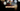# Leetcode | Solution of implement strStr() in JavaScript

March 30th, 2020
|

In this post, we will solve problem implement strStr() from leetcode and compute it's time and space complexities. Let's begin.

# Problem Statement

The question can be found at leetcode implement strStr() problem.

The problem states that we are given a haystack(big string) and a needle(small string). We need to find the first index of the needle in the haystack.

# Constraints and challenges

• Return `0` if the needle is empty
• Return `-1` if the needle is not present in the haystack
• Playing with string length in for loops can be tricky

# Solution

One funny solution is to use the `indexOf` method. But we can't use that as that's the method we need to implement.

The approach is very simple

• we will loop over haystack and for every index, check if the needle is equal to haystack substring from that index
• we can optimize this a bit as we don't need to loop till the end of the haystack, only till haystack length minus needle length as beyond that, there's not enough characters in the haystack to be equal to needle
• we will use the `substring` method and simple equality operations to write the logic in the loop body

We have discussed the approach, I urge you to go ahead on leetcode and give it another try.If you are here, it means something went wrong in implementation or you are just too lazy. In any case, let's see a simple implementation of the above logic.
``````var strStr = function (haystack, needle) {
if (needle.length === 0) return 0;

if (needle === haystack) return 0;

for (let i = 0; i <= haystack.length - needle.length; i++) {
if (needle === haystack.substring(i, i + needle.length)) {
return i;
}
}

return -1;
};``````

Let's look at the solution.

First, we handle two edge cases. If the needle is empty `return 0`. If the needle is equal to the haystack, no point in searching the haystack, so we `return 0` i.e. the index in the haystack where the needle exists.

Next, we loop over the haystack but notice the condition in for loop. We are only looping till `haystack.length - needle.length` because as discussed above, that's the last index where you can have a needle. In the body, we are checking for the equality of needle and haystack substring.

In the end, if we could not find any match we `return -1`.

On submission, here are the stats

``````Status: Accepted
Runtime: 52ms
Memory: 34MB``````

## Time and space complexity

### Time complexity

We are using a simple for loop to loop over the haystack, however, we are also using a substring method inside the loop with also has linear complexity, so complexity becomes O(n*m). where n is the length of haystack and m is the length of the needle

### Space complexity

We are not using extra space, thus, space complexity would be O(1).

# Summary

So, we solved the implement strStr() using a simple loop and substring method and finally, calculated the time and space complexities.

I hope you enjoyed solving this question. This is it for this one, complete source code for this post can be found on my Github Repo. Will see you in the next one.